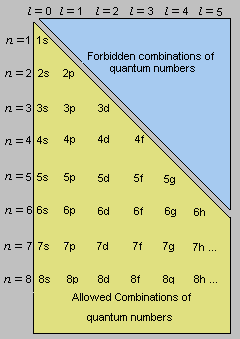# What electron could have quantum numbers n = 3, l = 0, m_l = 0, m_s = -1/2?

Mar 14, 2017

It would be a $\text{3s}$ electron.

#### Explanation:

The principal quantum number $n = 3$ tells us that the electron is in the third energy level.

The secondary quantum number $l = 0$ tells us the electron is an $\text{s}$ electron.The magnetic quantum number ${m}_{l}$ must be zero if $l = 0$.

The spin quantum number $s$ can be either +½ or -½.

∴ The electron is a $\text{3s}$ electron.